# End-to-End Bluetooth BR/EDR PHY Simulation with AWGN, RF Impairments and Corrections

This example shows an end-to-end simulation to measure the bit error rate (BER) and packet error rate (PER) for different Bluetooth® BR/EDR physical layer (PHY) packet types by using Bluetooth® Toolbox. These PHY packets are distorted by adding radio front-end (RF) impairments and the additive white Gaussian noise (AWGN). The distorted Bluetooth BR/EDR waveforms are processed at the practical receiver to get the BER and PER values. The obtained simulation results show the plots of BER and PER as a function of energy-to-noise density ratio (Eb/No).

Bluetooth is a short-range Wireless Personal Area Network (WPAN) technology, operating in the globally unlicensed industrial, scientific, and medical (ISM) band in the frequency range of 2.4 GHz to 2.485 GHz. In Bluetooth technology, data is divided into packets. Each packet is transmitted on one of the 79 designated Bluetooth channels. The bandwidth of each channel is 1 MHz. Bluetooth implements the frequency-hopping spread spectrum (FHSS) scheme to switch a carrier between multiple frequency channels by using a pseudorandom sequence known to the transmitter and the receiver.

The Bluetooth standard specifies these PHY modes:

Basic rate (BR) - Mandatory mode, uses Gaussian frequency shift keying (GFSK) modulation with a data rate of 1 Mbps.

Enhanced data rate (EDR) - Optional mode, uses phase shift keying (PSK) modulation with these two variants:

• EDR2M: Uses pi/4-DQPSK with a data rate of 2 Mbps

• EDR3M: Uses 8-DPSK with a data rate of 3 Mbps

This end-to-end Bluetooth BR/EDR PHY simulation determines BER and PER performance of one Bluetooth packet that has RF impairments and AWGN. Each packet is generated over a loop of a vector equal to length of the energy-to-noise density ratio (Eb/No) using the `bluetoothWaveformGenerator` function by configuring the `bluetoothWaveformConfig` object.

To accumulate the error rate statistics, the generated waveform is altered with RF impairments and AWGN before passing through the receiver.

These RF impairments are used to distort the packet:

• DC offset

• Carrier frequency offset

• Static timing offset

• Timing drift

White Gaussian noise is added to the generated Bluetooth BR/EDR waveforms. The distorted and noisy waveforms are processed through a practical Bluetooth receiver performing these operations:

• Remove DC offset

• Detect the signal bursts

• Perform matched filtering

• Estimate and correct the timing offset

• Estimate and correct the carrier frequency offset

• Demodulate BR/EDR waveform

• Perform forward error correction (FEC) decoding

• Perform data dewhitening

• Perform header error check (HEC) and cyclic redundancy check (CRC)

• Outputs decoded bits and decoded packet statistics based on decoded lower address part (LAP), HEC, and CRC

This block diagram illustrates the processing steps for each Bluetooth BR/EDR PHY packet.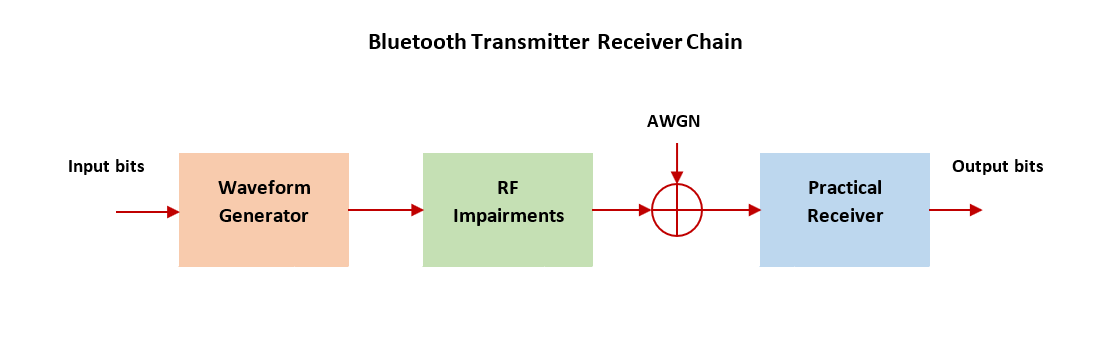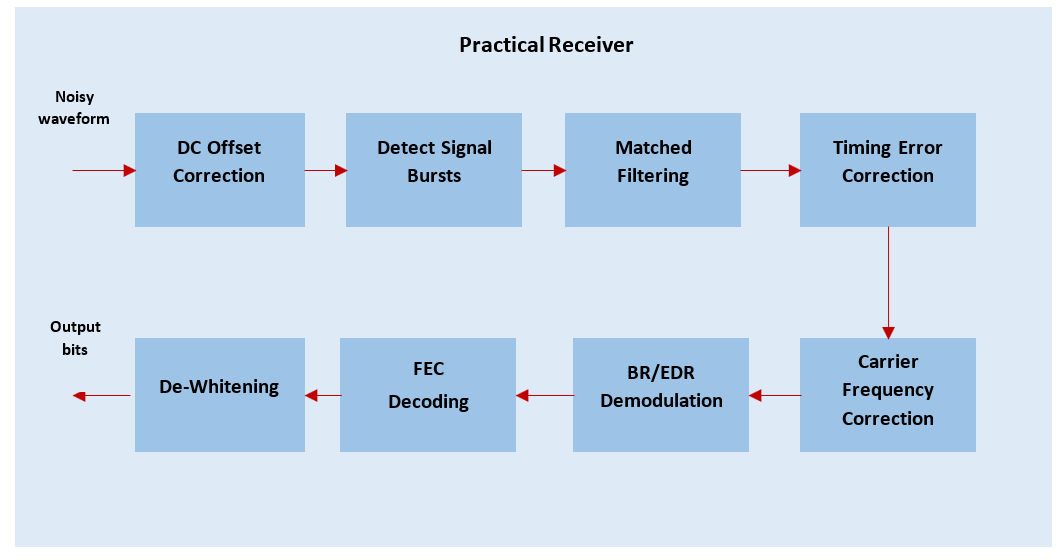To determine the BER and the PER, compare the recovered output bits with the transmitted data bits.

### Initialize Simulation Parameters

```% Eb/No in dB EbNo = 2:2:14; % Maximum number of bit errors simulated at each Eb/No point maxNumErrs = 100; % Maximum number of bits accumulated at each Eb/No point maxNumBits = 1e6; % Maximum number of packets considered at each Eb/No point maxNumPkts = 1000; ```

In this example, the values for maxNumErrs, maxNumBits, and maxNumPkts are selected for a short simulation time.

### Configure Bluetooth BR/EDR Waveform

The Bluetooth BR/EDR waveform is configured by using the `bluetoothWaveformConfig` object. Configure the properties of the `bluetoothWaveformConfig` object as per your requirements. In this example, the PHY mode of transmission, the Bluetooth packet type, and the number of samples per symbol are configured.

```phyMode =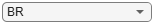'BR'; % PHY transmission mode bluetoothPacket = 'FHS'; % Type of Bluetooth packet, this value can be: {'ID', % 'NULL','POLL','FHS','HV1','HV2','HV3','DV','EV3', % 'EV4','EV5','AUX1','DM3','DM1','DH1','DM5','DH3', % 'DH5','2-DH1','2-DH3','2-DH5','2-DH1','2-DH3', % '2-DH5','2-EV3','2-EV5','3-EV3','3-EV5'} sps = 8; % Samples per symbol, must be greater than 1 ```

### Configure RF Impairments

Set frequency, time, and DC offset parameters to distort the Bluetooth BR/EDR waveform.

```frequencyOffset =6000;% In Hz timingOffset =0.5; % In samples, less than 1 microsecond timingDrift =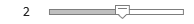2; % In parts per million dcOffset =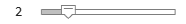2; % Percentage w.r.t maximum amplitude value symbolRate = 1e6; % Symbol Rate % Create timing offset object timingDelayObj = dsp.VariableFractionalDelay; % Create frequency offset object frequencyDelay = comm.PhaseFrequencyOffset('SampleRate',symbolRate*sps);```

### Process Eb/No Points

For each Eb/No point, packets are generated and processed through these steps:

• Generate random bits

• Generate Bluetooth BR/EDR waveform

• Pass generated waveform through AWGN channel

• Pass distorted waveform through practical receiver

• Calculate BER and PER

```ber = zeros(1,length(EbNo)); % BER results per = zeros(1,length(EbNo)); % PER results bitsPerByte = 8; % Number of bits per byte % Set code rate based on packet if any(strcmp(bluetoothPacket,{'FHS','DM1','DM3','DM5','HV2','DV','EV4'})) codeRate = 2/3; elseif strcmp(bluetoothPacket,'HV1') codeRate = 1/3; else codeRate = 1; end % Set number of bits per symbol based on the PHY transmission mode bitsPerSymbol = 1+ (strcmp(phyMode,'EDR2M'))*1 +(strcmp(phyMode,'EDR3M'))*2; % Get SNR from EbNo values snr = EbNo + 10*log10(codeRate) + 10*log10(bitsPerSymbol) - 10*log10(sps); % Create a Bluetooth BR/EDR waveform configuration object txCfg = bluetoothWaveformConfig('Mode',phyMode,'PacketType',bluetoothPacket,... 'SamplesPerSymbol',sps); if strcmp(bluetoothPacket,'DM1') txCfg.PayloadLength = 17; % Maximum length of DM1 packets in bytes end dataLen = getPayloadLength(txCfg); % Length of the payload % Get PHY properties rxCfg = getPhyConfigProperties(txCfg); for iSnr = 1:length(snr) rng default % Initialize error computation parameters errorCalc = comm.ErrorRate; berVec = zeros(3,1); pktCount = 0; % Counter for number of detected Bluetooth packets loopCount = 0; % Counter for number of packets at each SNR value pktErr = 0; while((berVec(2) < maxNumErrs) && (berVec(3) < maxNumBits) && (loopCount < maxNumPkts)) txBits = randi([0 1],dataLen*bitsPerByte,1); % Data bits generation txWaveform = bluetoothWaveformGenerator(txBits,txCfg); % Add Frequency Offset frequencyDelay.FrequencyOffset = frequencyOffset; transWaveformCFO = frequencyDelay(txWaveform); % Add Timing Delay timingDriftRate = (timingDrift*1e-6)/(length(txWaveform)*sps);% Timing drift rate timingDriftVal = timingDriftRate*(0:1:(length(txWaveform)-1))';% Timing drift timingDelay = (timingOffset*sps)+timingDriftVal; % Static timing offset and timing drift transWaveformTimingCFO = timingDelayObj(transWaveformCFO,timingDelay); % Add DC Offset dcValue = (dcOffset/100)*max(transWaveformTimingCFO); txImpairedWaveform = transWaveformTimingCFO + dcValue; % Add AWGN txNoisyWaveform = awgn(txImpairedWaveform,snr(iSnr),'measured'); % Receiver Module [rxBits,decodedInfo,pktStatus]... = helperBluetoothPracticalReceiver(txNoisyWaveform,rxCfg); numOfSignals = length(pktStatus); pktCount = pktCount+numOfSignals; loopCount = loopCount+1; % BER and PER Calculations L1 = length(txBits); L2 = length(rxBits); L = min(L1,L2); if(~isempty(L)) berVec = errorCalc(txBits(1:L),rxBits(1:L)); end pktErr = pktErr+sum(~pktStatus); end % Average of BER and PER per(iSnr) = pktErr/pktCount; ber(iSnr) = berVec(1); if ((ber(iSnr) == 0) && (per(iSnr) == 1)) ber(iSnr) = 0.5; % If packet error rate is 1, consider average BER of 0.5 end if ~any(strcmp(bluetoothPacket,{'ID','NULL','POLL'})) disp(['Mode ' phyMode ', '... 'Simulated for Eb/No = ', num2str(EbNo(iSnr)), ' dB' ', '... 'obtained BER:',num2str(ber(iSnr)),' obtained PER: ',... num2str(per(iSnr))]); else disp(['Mode ' phyMode ', '... 'Simulated for Eb/No = ', num2str(EbNo(iSnr)), ' dB' ', '... 'obtained PER: ',num2str(per(iSnr))]); end end```
```Mode BR, Simulated for Eb/No = 2 dB, obtained BER:0.24074 obtained PER: 1 Mode BR, Simulated for Eb/No = 4 dB, obtained BER:0.087191 obtained PER: 1 Mode BR, Simulated for Eb/No = 6 dB, obtained BER:0.063492 obtained PER: 0.86667 Mode BR, Simulated for Eb/No = 8 dB, obtained BER:0.025 obtained PER: 0.77419 Mode BR, Simulated for Eb/No = 10 dB, obtained BER:0.0083333 obtained PER: 0.38824 Mode BR, Simulated for Eb/No = 12 dB, obtained BER:0.0019597 obtained PER: 0.13699 Mode BR, Simulated for Eb/No = 14 dB, obtained BER:0.00025304 obtained PER: 0.022267 ```

### Simulation Results

This section presents the BER and PER results with respect to the input Eb/No range for the considered PHY mode.

```figure, if any(strcmp(bluetoothPacket,{'ID','NULL','POLL'})) numOfPlots = 1; % Plot only PER else numOfPlots = 2; % Plot both BER and PER subplot(numOfPlots,1,1),semilogy(EbNo,ber.','-r*'); xlabel('Eb/No (dB)'); ylabel('BER'); legend(phyMode); title('BER of Bluetooth with RF impairments'); hold on; grid on; end subplot(numOfPlots,1,numOfPlots),semilogy(EbNo,per.','-k*'); xlabel('Eb/No (dB)'); ylabel('PER'); legend(phyMode); title('PER of Bluetooth with RF impairments'); hold on; grid on;```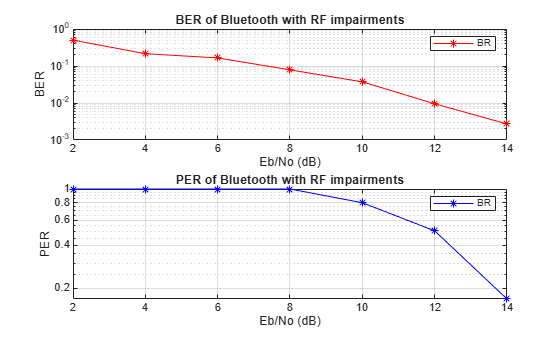### Appendix

The example uses this helper function:

This example shows an entire end-to-end procedure to generate a Bluetooth BR/EDR packet. The generated Bluetooth BR/EDR waveform is distorted by adding RF impairments and AWGN. To get the BER and PER values, the distorted Bluetooth BR/EDR waveform is synchronized, demodulated, and decoded from the practical receiver.

### Selected Bibliography

1. Bluetooth Special Interest Group (SIG). "Core System Package [BR/EDR Controller Volume]". Bluetooth Core Specification. Version 5.3, Volume 2. www.bluetooth.com